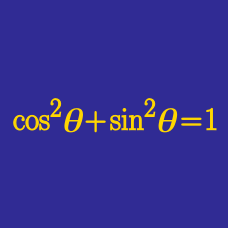Geometry

# Ratio of Trigonometric Functions

Which of the following is equal to

$\frac{ \sin \theta } { \cos \theta} ?$

If $$\displaystyle \sin \theta=\frac{15}{17},$$ what is the value of $\cos \theta \times \tan \theta ?$

Which of the following is equal to

$\frac{ \cot \theta } { \cos \theta} ?$

If $$\cot \theta=\frac{24}{19},$$ what is the value of $\frac{\sec \theta}{\csc \theta} ?$

If $$\displaystyle \tan \theta=\frac{20}{19},$$ what is the value of $\frac{\cos \theta}{\sin \theta}?$

×

Problem Loading...

Note Loading...

Set Loading...